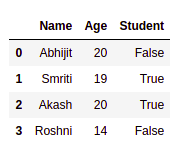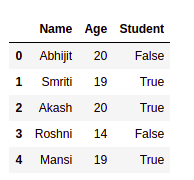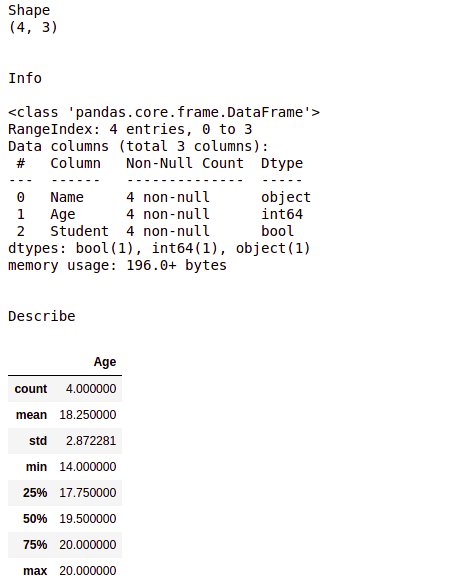Skip to content
Related Articles
Data Manipulattion in Python using Pandas
• Last Updated : 10 May, 2020

In Machine Learning, the model requires a dataset to operate, i.e. to train and test. But data doesn’t come fully prepared and ready to use. There are discrepancies like “Nan”/ “Null” / “NA” values in many rows and columns. Sometimes the data set also contains some of the row and columns which are not even required in the operation of our model. In such conditions, it requires proper cleaning and modification of the data set to make it an efficient input for our model. We achieve that by practicing “Data Wrangling” before giving data input to the model.

Ok, So let’s dive into the programming part. Our first aim is to create a Pandas dataframe in Python, as you may know, pandas is one of the most used libraries of Python.

Example:

 `# importing the pandas library``import` `pandas as pd`` ` ` ` `# creating a dataframe object``student_register ``=` `pd.DataFrame()`` ` `# assigning values to the ``# rows and columns of the``# dataframe``student_register[``'Name'``] ``=` `[``'Abhijit'``, ``                            ``'Smriti'``,``                            ``'Akash'``,``                            ``'Roshni'``]`` ` `student_register[``'Age'``] ``=` `[``20``, ``19``, ``20``, ``14``]``student_register[``'Student'``] ``=` `[``False``, ``True``,``                               ``True``, ``False``]`` ` `student_register`

Output:As you can see, the dataframe object has four rows `[0, 1, 2, 3]` and three columns`[“Name”, “Age”, “Student”]` respectively. The column which contains the index values i.e. [0, 1, 2, 3] is known as the index column, which is a default part in pandas datagram. We can change that as per our requirement too because Python is powerful.
Next, for some reason we want to add a new student in the datagram, i.e you want to add a new row to your existing data frame, that can be achieved by the following code snippet.

One important concept is that the “dataframe” object of Python, consists of rows which are “series” objects instead, stack together to form a table. Hence adding a new row means creating a new series object and appending it to the dataframe.

Example:

 `# creating a new pandas``# series object``new_person ``=` `pd.Series([``'Mansi'``, ``19``, ``True``], ``                       ``index ``=` `[``'Name'``, ``'Age'``, ``                                ``'Student'``])`` ` `# using the .append() function``# to add that row to the dataframe``student_register.append(new_person, ignore_index ``=` `True``)`

Output:Before processing and wrangling any data you need to get the total overview of it, which includes statistical conclusions like standard deviation(std), mean and it’s quartile distributions. Also, you need to know the exact information of each column, i.e. what type of value it stores and how many of them are unique. There are three support functions, `.shape`, `.info()` and `.describe()`, which outputs the shape of the table, information on rows and columns, and statistical information of the dataframe (numerical column only) respectively.

Example:

 `# for showing the dimension ``# of the dataframe``print``(``'Shape'``)``print``(student_register.shape)`` ` `# showing info about the data ``print``(``"\n\nInfo\n"``)``student_register.info()`` ` `# for showing the statistical ``# info of the dataframe``print``(``"\n\nDescribe"``)``student_register.describe()`

Output:In the above example, the `.shape` function gives an output (4, 3) as that is the size of the created dataframe.

The description of the output given by `.info()` method is as follows:

1. “RangeIndex” describes about the index column, i.e. [0, 1, 2, 3] in our datagram. Which is the number of rows in our dataframe.
2. As the name suggests “Data columns” give the total number of columns as output.
3. “Name”, “Age”, “Student” are the name of the columns in our data, “non-null ” tells us that in the corresponding column, there is no NA/ Nan/ None value exists. “object”, “int64″ and “bool” are the datatypes each column have.
4. “dtype” gives you an overview of how many data types present in the datagram, which in term simplifies the data cleaning process.
Also, in high-end machine learning models, “memory usage” is an important term, we can’t neglect that.

The description of the output given by `.describe()` method is as follows:

1. count is the number of rows in the dataframe.
2. mean is the mean value of all the entries in the “Age” column.
3. std is the standard deviation of the corresponding column.
4. min and max are the minimum and maximum entry in the column respectively.
5. 25%, 50% and 75% are the First Quartiles, Second Quartile(Median) and Third Quartile respectively, which gives us important info on the distribution of the dataset and makes it simpler to apply an ML model.

Attention geek! Strengthen your foundations with the Python Programming Foundation Course and learn the basics.

To begin with, your interview preparations Enhance your Data Structures concepts with the Python DS Course. And to begin with your Machine Learning Journey, join the Machine Learning – Basic Level Course

My Personal Notes arrow_drop_up